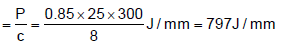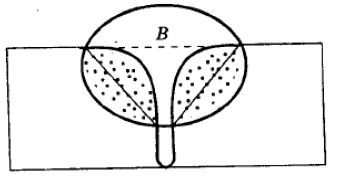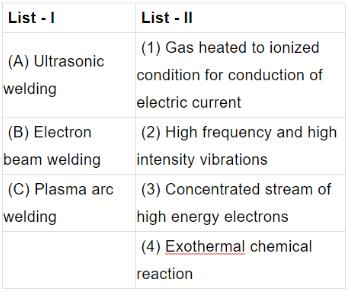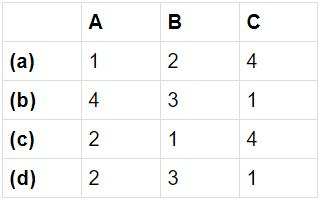Courses

# Fabrication Processes (Welding) - MCQ Test 2

## 30 Questions MCQ Test Mock Test Series - Mechanical Engineering (ME) for GATE 2020 | Fabrication Processes (Welding) - MCQ Test 2

Description
This mock test of Fabrication Processes (Welding) - MCQ Test 2 for Mechanical Engineering helps you for every Mechanical Engineering entrance exam. This contains 30 Multiple Choice Questions for Mechanical Engineering Fabrication Processes (Welding) - MCQ Test 2 (mcq) to study with solutions a complete question bank. The solved questions answers in this Fabrication Processes (Welding) - MCQ Test 2 quiz give you a good mix of easy questions and tough questions. Mechanical Engineering students definitely take this Fabrication Processes (Welding) - MCQ Test 2 exercise for a better result in the exam. You can find other Fabrication Processes (Welding) - MCQ Test 2 extra questions, long questions & short questions for Mechanical Engineering on EduRev as well by searching above.
QUESTION: 1

Solution:
QUESTION: 2

Solution:
QUESTION: 3

### By which one of the following methods gray iron is usually welded?

Solution:
QUESTION: 4

Preheating before welding is done to

Solution:
QUESTION: 5

In a gas welding of mild steel using an oxy-acetylene flame, the total amount of acetylene consumed is 10 litre. The oxygen consumption from the cylinder is

Solution:
QUESTION: 6

In arc welding, d.c. reverse polarity is used to hear greater advantage in

Solution:
QUESTION: 7

In an arc welding process, the voltage and current are 25 V and 300 A respectively. The arc heat transfer efficiency is 0.85 and welding speed is 8 mm/see. The net heat input (in J/mm) is

Solution:

Effective heat transfer per second (P) = η VI =0.85x25x300 J/s

Speed (c) = 8 mm/s

Net heat inputQUESTION: 8

Arc blow is more common in

Solution:
QUESTION: 9

An arc welded joint is shown in the above figure. The part labelled 'B' in the figure is known asSolution:
QUESTION: 10

In which of the following welding processes, flux is used in the form of granules?

Solution:
QUESTION: 11

In resistance welding, heat is generated due to the resistance between

Solution:
QUESTION: 12

Assertion (A): Spot welding is adopted to weld two overlapped metal pieces between two electrode points. Reason (R): In this process when current is switched on, the lapped pieces of metal are heated in a restricted area.

Solution:
QUESTION: 13

Two 1 mm thick steel sheets are to be spot welded at a current of 5000 A. Assuming effective resistance to be 200 micro-ohms and current flow time of 0.2 second, heat generated during the process will be

Solution:

Q = I2Rt = 50002 × (200 ×10−6 ) × 0.2J = 1000J

QUESTION: 14

Assertion (A): In electron beam welding process, vacuum is an essential process parameter

Reason (R): Vacuum provides a highly efficient shield on weld zone

Solution:
QUESTION: 15

Match List I (Welding processes) with List II (Features) and select the correct answer using the codes given below the Lists:Codes:Solution:
QUESTION: 16

Which one of the following is a solid state joining process?

Solution:
QUESTION: 17

The strength of a brazed joint

Solution:
QUESTION: 18

Consider the following statements: The magnitude of residual stresses in welding depends upon

1. design of weldment

2. support and clamping of components

3. welding process used

4. amount of metal melted / deposited

Which of the statements given above are correct?

Solution:
QUESTION: 19

The ratio of acetylene to oxygen is approximately………. for a neutral flames used in gas welding.

Solution:
QUESTION: 20

In Oxyacetylene gas welding, temperature at the inner cone of the flame is around

Solution:
QUESTION: 21

In oxy-acetylene gas welding, for complete combustion, the volume of oxygen required per unit of acetylene is

Solution:
QUESTION: 22

Oxyacetylene reducing flame is used while carrying out the welding on

Solution:
QUESTION: 23

Assertion (A): The electrodes of ac arc welding are coated with sodium silicate, whereas electrodes used for dc arc welding are coated with potassium silicate binders.

Reason (R): Potassium has a lower ionization potential than sodium.

Solution:
QUESTION: 24

In d.c. welding, the straight polarity (electrode negative) results in

Solution:
QUESTION: 25

Which one of the following statements is correct?

Solution:
QUESTION: 26

In MIG welding, the metal is transferred into the form of which one of the following?

Solution:
QUESTION: 27

For butt -welding 40 mm thick steel plates, when the expected quantity of such jobs is 5000 per month over a period of 10 year, choose the best suitable welding process out of the following available alternatives.

Solution:

We need maximum metal deposition rate so choice SAW.
Note: You know maximum metal deposition rate of all welding is SAW and it may joint 40 mm thick plates easily.

QUESTION: 28

Resistance spot welding is performed on two plates of 1.5 mm thickness with 6 mm diameter electrode, using 15000 A current for a time duration of 0.25 seconds. Assuming the interface resistance to be 0.0001 Ω , the heat generated to form the weld is

Solution:

H = I2Rt = 150002 × 0.0001× 0.25J = 5625 W − s

QUESTION: 29

Which one of the following is not an electric resistance method of welding?

Solution:
QUESTION: 30

In which one of the following welding techniques is vacuum environment required?

Solution: